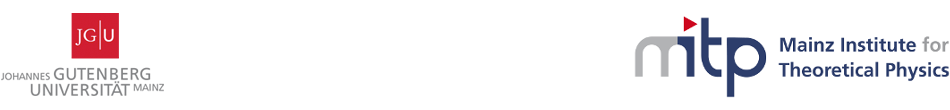#Geometry, Gravity and Supersymmetry

24-28 April 2017
Mainz Institute for Theoretical Physics, Johannes Gutenberg University
Europe/Berlin timezone

## Compact pseudo-Riemannian homogeneous Einstein manifolds of low dimension

26 Apr 2017, 16:30
30m
02.430 (Mainz Institute for Theoretical Physics, Johannes Gutenberg University)

### 02.430

#### Mainz Institute for Theoretical Physics, Johannes Gutenberg University

Staudingerweg 9 / 2nd floor, 55128 Mainz

### Speaker

Dr Wolfgang Globke (University of Adelaide)

### Description

Let $M$ be pseudo-Riemannian homogeneous Einstein manifold of finite volume, and suppose a connected Lie group $G$ acts transitively and isometrically on $M$. We study such spaces $M$ in three important cases. First, we assume $\langle\cdot,\cdot\rangle$ is invariant, in which case the Einstein property requires that $G$ is either solvable or semisimple. Next, we investigate the case where $G$ is solvable. Here, $M$ is compact and $M=G/\Gamma$ for a lattice $\Gamma$ in $G$. We show that in dimensions less or equal to $7$, compact quotients $M=G/\Gamma$ exist only for nilpotent groups $G$. We conjecture that this is true for any dimension. In fact, this holds if Schanuel's Conjecture on transcendental numbers is true. Finally, we consider semisimple Lie groups $G$, and find that $M$ splits as a pseudo-Riemannian product of Einstein quotients for the compact and the non-compact factors of $G$. This is joint work with Yuri Nikolayevsky (La Trobe University).

### Primary author

Dr Wolfgang Globke (University of Adelaide)

### Co-author

Prof. Yuri Nikolayevsky (La Trobe University)

 Slides